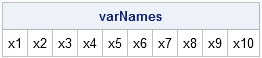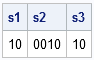Statistical programmers can be creative and innovative. But when it comes to choosing names of variables, often x1, x2, x3,... works as well as any other choice. In this blog post, I have two tips that are related to constructing variable names of the form x1, x2,..., xn. Both tips are from my book, Statistical Programming with SAS/IML Software.

### Constructing Variable Names with a Common Prefix

The SAS/IML language supports the index creation operator (:), which enables you to create variable names with a common prefix and a numerical suffix. For example, suppose that you want to create the character vector {"x1" "x2" ... "x10"}. You can use the index creation operator, as shown in the following statements:

```proc iml; /** create variable names with sequential values **/ varNames = "x1":"x10"; print varNames;```Notice that the index creation operator creates a row vector that has 1 row and p columns.

This technique is useful for constructing column names for a SAS/IML matrix or for constructing variable names for data that you are writing to a SAS data set.

Tip: Use the index creation operator (:) to create a vector of names with a common prefix and a numerical suffix (for example, "x1":"x10").

### Constructing a Variable Name by Concatenating a Prefix and Suffix

The previous example creates 10 variable names. But how can you handle the case where the maximal value (10) is not known but is contained in a scalar SAS/IML matrix? For example, if your data are in a matrix, x, you might use the NCOL function to count the number of columns in the matrix, as shown in the following statement:

`p = ncol(x);`

At the time that the statement is written, the programmer does not know the value of p. It might be 5, it might be 100.

You can use the CHAR function or the PUTN function to convert the value of p into a character string. Both of these functions apply a SAS format to the value of p. The CHAR function applies the w.d format, whereas the PUTN function applies a format that you specify. For example, if you know that the value of p is between 0 and 9,999 (that is, can be represented in four or fewer digits), either of the following statements convert p to a character string:

```/** convert to character string **/ s1 = char(p, 4); /** apply w.d format **/ s2 = putn(p, "Z4."); /** apply Zw.d format **/ s3 = putn(p, "BEST4."); /** apply BESTw. format **/ print s1 s2 s3;```You can use the string concatenation operator (+) or the CONCAT function to concatenate this suffix onto a root string, such as "x." However, the strings s1 and s3 have leading blanks, even though they are not visible in the displayed output. You can use the STRIP function to remove them, as shown in the following statements:

```endName = "x" + strip(s1); varNames = "x1":endName;```

Tip: When concatenating character strings, use the STRIP function to remove trailing and leading blanks, as shown in the following statement:
`endName = "x" + strip(char(p,4));`

The syntax strip(char(...)) is extremely useful, and should be part of every statistical programmer's toolbox. When you need to form a suffix that is not an integer, use the general syntax strip(putn(...)).

ShareDistinguished Researcher in Computational Statistics

Rick Wicklin, PhD, is a distinguished researcher in computational statistics at SAS and is a principal developer of SAS/IML software. His areas of expertise include computational statistics, simulation, statistical graphics, and modern methods in statistical data analysis. Rick is author of the books Statistical Programming with SAS/IML Software and Simulating Data with SAS.

1.Chris Peters on

Is it possible to do a two part convention?

For example:

x1y1:x100y100

x1y1 x1y2 x1y3 ..... x100y100

2.Rick Wicklin on

Not automatically. But for your example you can create two arrays of strings and then concatenate them together. For example:
s = 'x1':'x10';
r = 'y1':'y10';
c = cats(s,r);
print c;

3.Chris Peters on

That's almost it except that that code matches indexes of each 'x' and 'y'. Below are successive 'do' loops that I've used to do the job, but I'm still trying to figure out how to input each 'varNames' into a matrix and eventually append that to some dataset as column names.

Proc IML;
Reset Log Print;
Do i = 1 to 5;
names1 = cat("x",putn(i, "BEST4."));
Do t = 1 to 5;
names2 = cat("y",putn(t, "BEST4."));
varNames = cat(names1,names2);
End;
End;
Quit;

4.Chris Peters on

I solved it! Thanks! The code below is pretty inefficient. I think I may try to apply your blog post above about pre-defining matrices outside of loops.

Proc IML;
Reset Log Print;
Do i = 1 to 25;
If i < 10 then names1 = left(cat("x",putn(i, "BEST1.")));
else names1 = left(cat("x",putn(i, "BEST2.")));
Do t = 1 to 5;
If t < 10 then names2 = left(cat("y",putn(t, "BEST1.")));
else names2 = left(cat("y",putn(t, "BEST2.")));
varNames = varNames || cat(x,y);
End;
End;
Create Work.dataset from varNames[colname= varNames];
Append from varNames;
Quit;

5.Rick Wicklin on

There is a whole community of IML users at http://communities.sas.com/index.jspa

After you "register" and "create a profile," you can ask and get answers to questions like this. See you there!